The Math Colloquium Series Presents
Martin Flashman
Department of Mathematics
Humboldt State University
Mathematics Beyond Good and Evil: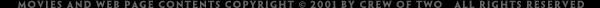in Geometry, Algebra, and Calculus!
Work in Progress
Thursday, November 21

Abstract: Duality in common language comes from the word "dual" meaning consisting of two parts or elements or having two like parts. Frequently duality refers to pairs that are opposites or complementary, such as good and evil, black and white, masculine and feminine, or yin and yang. Broadly speaking, mathematicians use the term duality to describe a pairing of concepts, objects, or numbers that have some features that are opposite or complementary. These dualities are found in geometry, algebra and many other mathematical subjects.
Professor Flashman will explore some related examples of mathematical duality from algebra, linear algebra, geometry, graph theory, and calculus. Though most of the presentation will not rely on any specific background, some familiarity with vectors and matrices will be useful for one or two of the examples.

1. Introduction
1.  MathSearch
2. Examples:
1. good and evil, black and white, masculine and feminine, or yin and yang.
2. right/left, short/tall, up/down, positive/negative, soft/hard, secular/sacred, smart/dumb,
3. Hildreth, P.J. & Kimble, C. (2002) "The duality of knowledge"Information Research, 8(1)
1.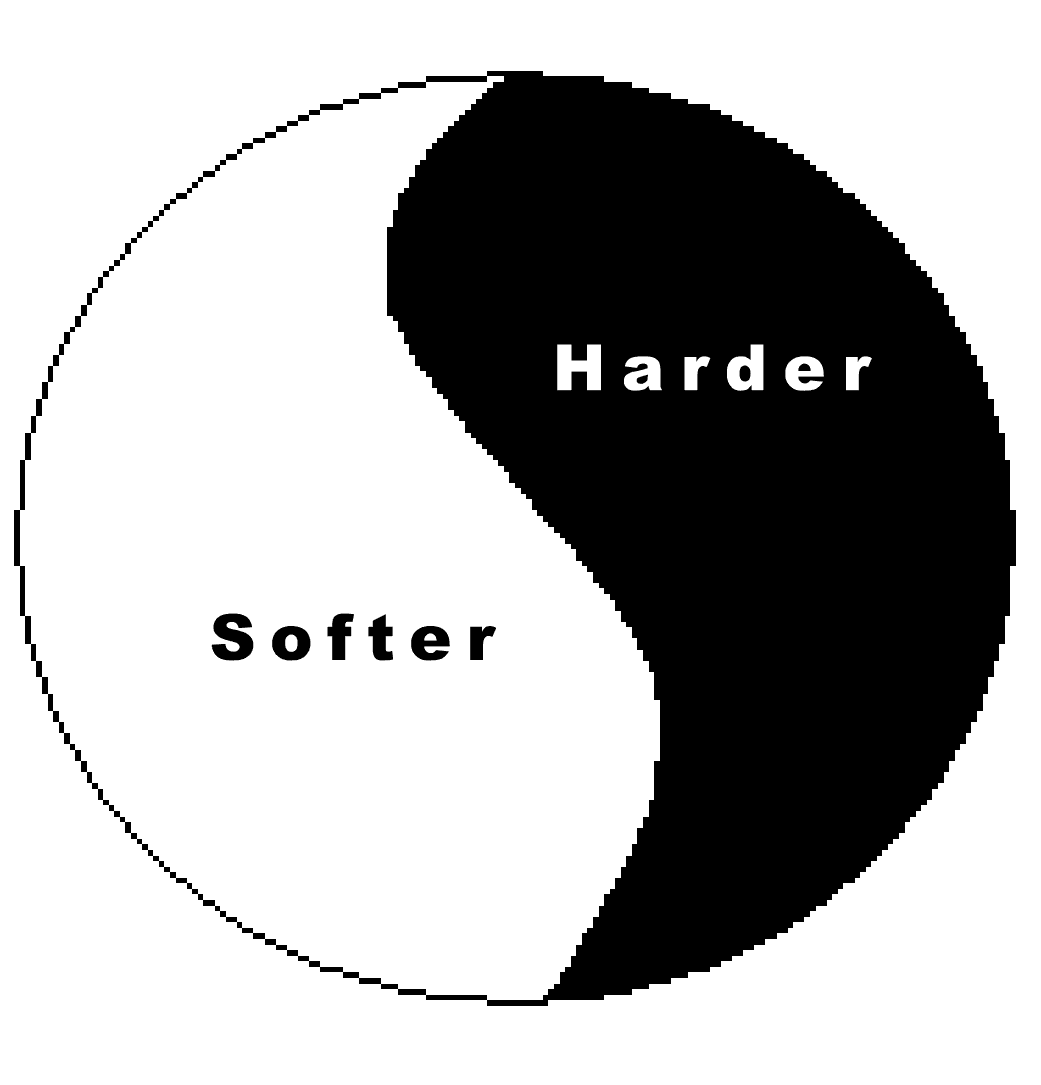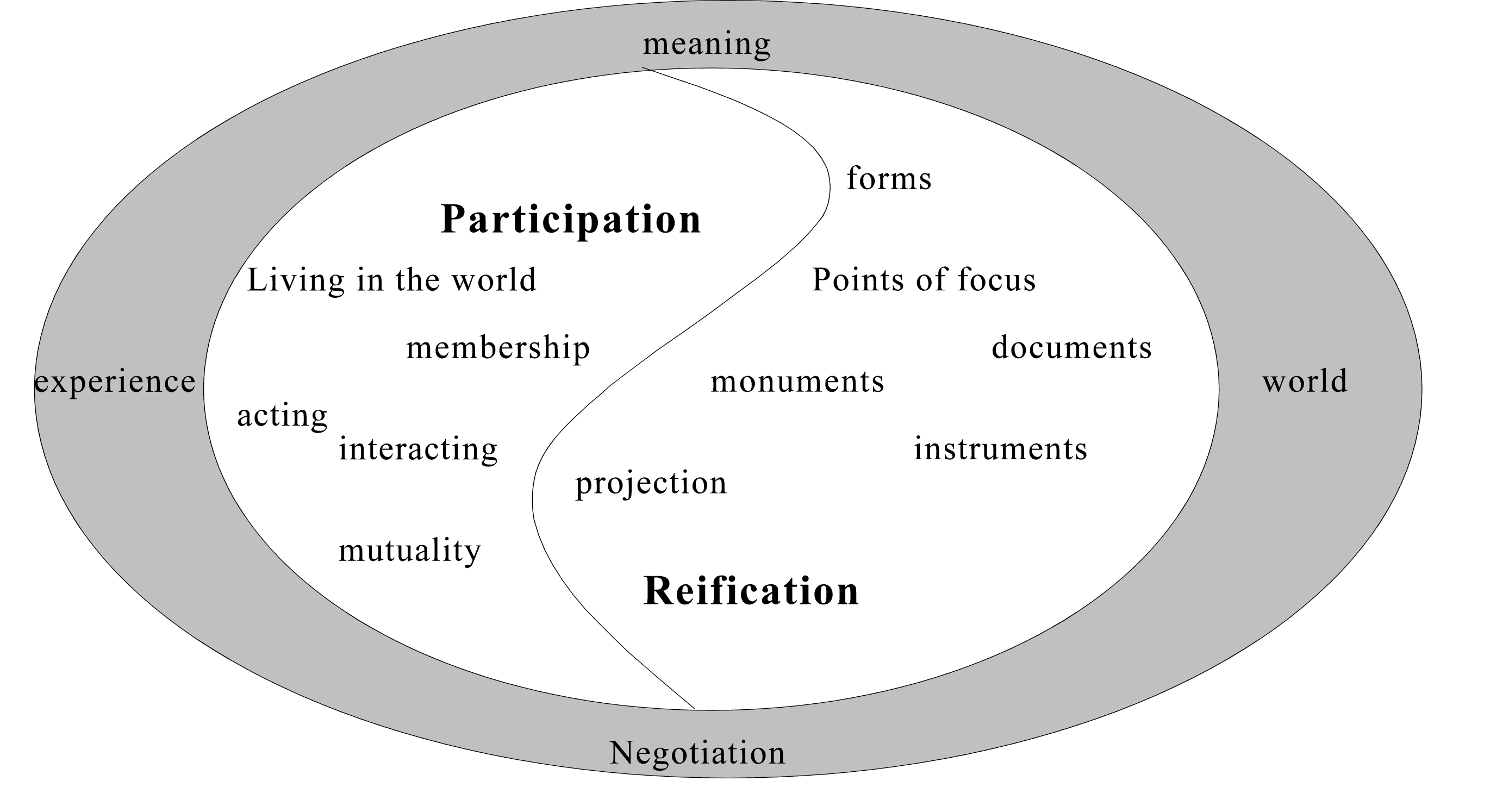4. Integer Programming: Facets, Subadditivity, and Duality for Group and Semi-group Problems (SIAM)
5. Conjugate Duality and Optimization
6. Finite Element Methods for Partial Differential Equations
7. Graph Mappings and Poincare Duality
8. Wave Particle Duality
9. "One relatively simple but elegant example is the duality between the Heterotic string compactified on T4 and the type IIA string compactified on a K3 manifold."

2. Duality in graphical configurations in the plane I: points and lines.
1. The triangle and n-gons.

2. [Use Wingeom]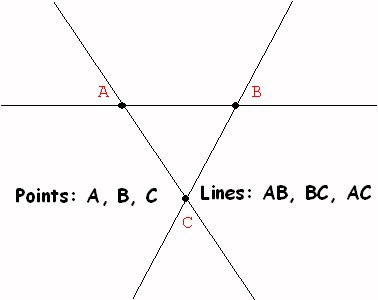3 points - 3 lines.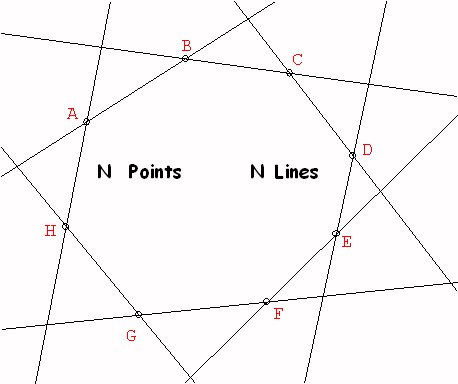N points - N lines.

1. Other popular configurations: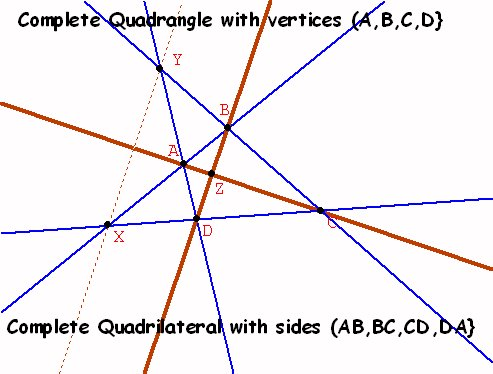The Complete Quadrangle:  4 points {A,B,C,D} determine 6 lines {AB,AC,AD, BC, BD, CD}  and three additional points {X,Y,Z}.The Complete Quadilateral:  4 lines {AB, BC, CD,AD}determine 6 points {A,B, C, D, X,Y}  with three additional lines{AC, BD, XY} .

1. The 7-7 Configuration.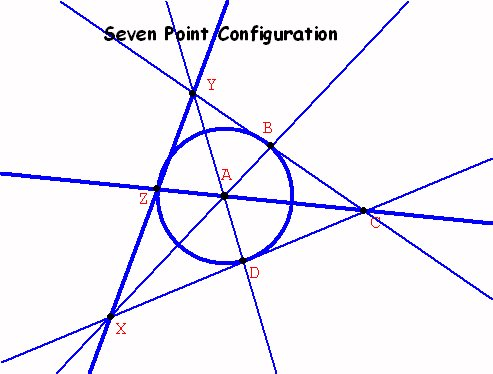3 points on each "line", 3 "lines" through each point
1. Desargues 10-10 Configuration: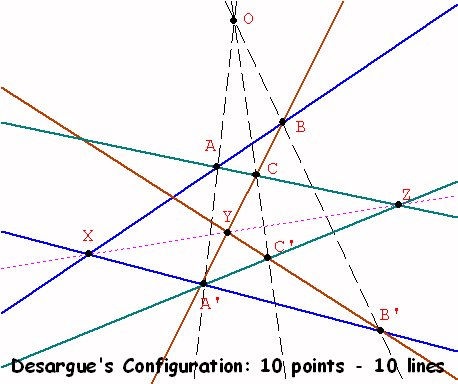Planar Configuration

 3 points on each line 3 lines through each point Triangles in Perspective  from a point Triangles in Perpective from a line

Desargue's Spatial Configuration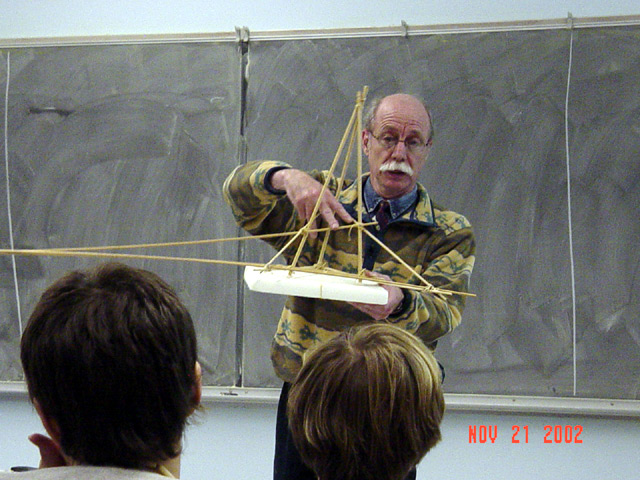1. Pascal Configuration and [the dual] Brianchon Configuration.
2.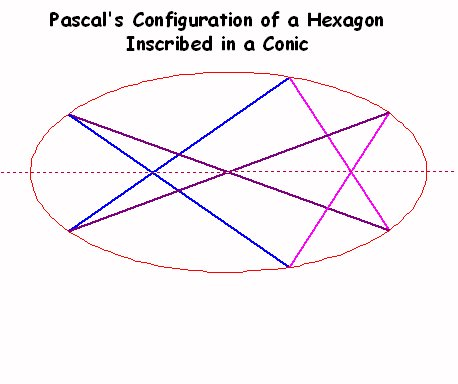Pascal: 9 points - 7 lines A Point Conic- The conic is described by a set of points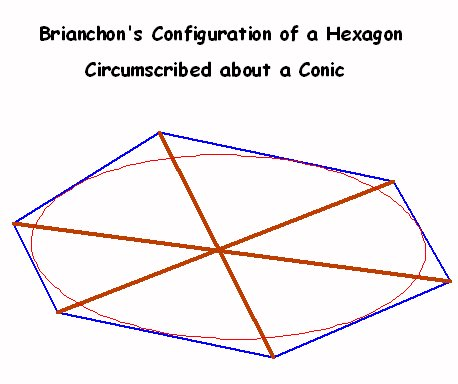Brianchon: 9 lines - 7 points A Line Conic-The conic is described by a set of lines

1. Duality in planar and spherical graphical configurations II : points and faces.
1. City - states. Borders - Highways.
2. "The dual  map"
1. How to build the dual map.

2. Replace a region with a dual vertex in that region.
Every country has a capital city.

Between neighboring regions add an edge crossing the original border connecting the dual vertices.
Every capital has a highway directly connecting it to neighboring capital.

Every vertex will be surrounded by a network of dual edges enclosing it in a dual region.

The dual map is made up of the dual vertices, dual edges , and the resulting dual regions.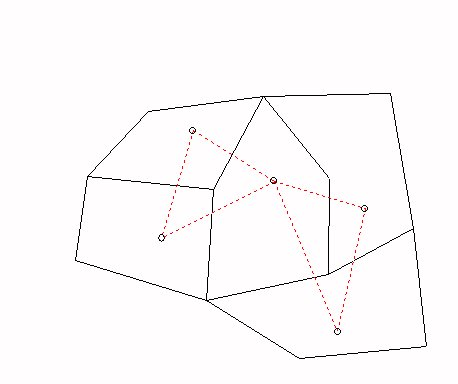Map in black. Dual Map in red.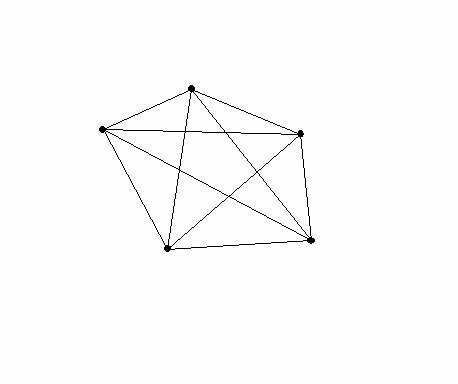Complete graph on 5 Vertices: 5Vertices - 10 Edges

3. Application: Given  5 planar regions, there must be at least two regions that do not have a common border.

4. Proof:
Suppose every pair of regions has a common border.
Consider the dual planar map.

This would have five vertices and each vertex would be connected to every other vertex by an edge ... a complete graph on 5 vertices.

But this graph would have 5 vertices and 10 edges and by Euler's formula* (Not proven here!) that

V - E  + R =  2
where R is the number of regions formed in the plane by the  graph, we have R =  2+E-V = 7.

Thus there must be 7 regions, and
each region would have at least 3 edges.
So if we count the edges (twice - once for each region on the edge) we would have at least 21 "edges" or at least 11 edges without double counting.
Which is a contradiction, since there are just 10 edges.

1. Duality and Polyhedra     duality

2. [Use Wingeom and models]
1. Cube- Octahedron
2. Dodecahedron - Icosahedron
3. Tetrahedon - Tetrahedon
4. Here is a table showing the five platonic solids and the duality relation of Vertices, Edges, and Faces:

 V F E Cube 8 6 12 Octahedron 6 8 12 Icosahedron 12 20 30 Dodecahedron 20 12 30 Tetrahedron 4 4 6

1. Duality in Linear Pogramming: [Use Winplot]

2. In canonical form:

Primal linear program problem: Min{cx: x >= 0, Ax >= b}.
Example:

 Minimize: C = x + 2y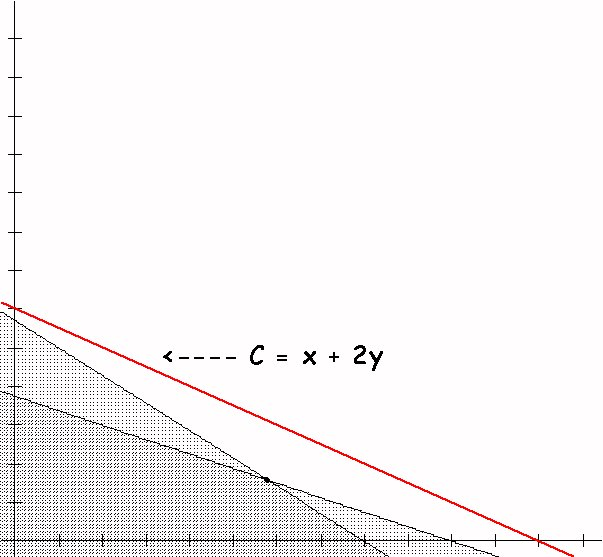5x + 7y >= 4 3x + 8y >= 3

Dual linear program problem:  Max{yb: y >= 0, yA <= c}.
Dual Example:

 Maximize: P = 4x + 3y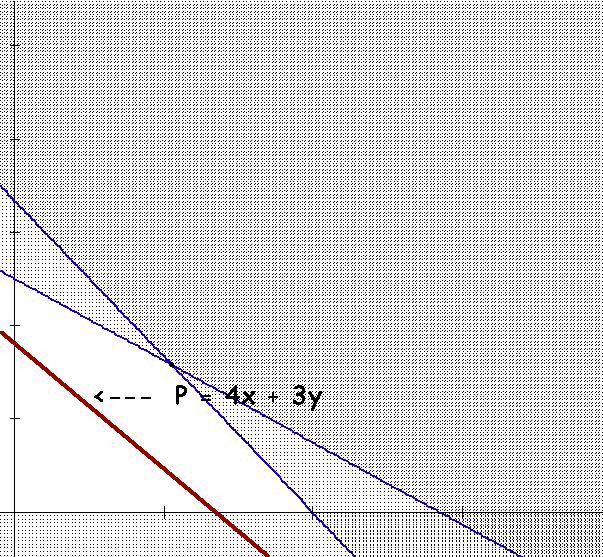5x + 3y <= 1 7x + 8y <= 2

Gale, Kuhn and Tucker (1951) proved the fundamental strong duality theorem:
Duality Theorem of Linear Programming.
For an LP primal-dual pair, exactly one of the following holds:
1. The primal has an optimal solution, in which case so must the dual, and their objective values are equal.

2. The primal is infeasible, in which case the dual is either infeasible or unbounded.

3. The primal is unbounded, in which case the dual is infeasible.

1. Duality in algebra/ geometry:
1. vector  as point, vector as transformation
1. v = <1, 6>  a position vector corresponding to the point (1,6) in  the Cartesian plane.
2. v  =  <1,6> as a translation of the plane. <1,6> (a,b) = (a+1, b+6).

3.
2. vector as a point, vector as a linear function(al). T: R2 -> R
1. v = <1,6> as before.
2. v = <1,6> ,  Tv(<x.y>)= <1,6> <x,y> = 1x+6y.   The dot product.
3. Theorem: The set of all linear functionals from Rn to R is an n dimensional vector space- called the dual space of Rn.

4. A basis for this vector space is given by the n projection functionals, pk(< x1, x2, ...,xk,...xn>) = xk or
pk = T<0,0,0,1,0...,0> for k = 1, 2,...,n.
3. Non zero vector as direction at the point (0,0,0), non zero vector as plane through (0,0,0) in R3.
1.  v = <1,6,2> = {(x,y,z) where x =t, y = 6t, z=2t and t is not  0.}.
2. v = [1,6,2] = { <x,y,z> where 1x + 6 y + 2 z = 0}
3.
4. Note the duality : In R3, [1,6,2] is the plane through the origin that is perpendicular to the direction <1,6,2>

5.  In R3, <1,6,2> is the direction  at  the origin that is perpendicular to the plane [1,6,2].
6. Note in this notation [1,6,2] = [5, 30, 10] = [ a, 6a, 2a] for any a not equal to 0.
7. Note if <1,6,2> is on [ a,b,c] if and only if <t,6t,2t> is also on [a,b,c] for any t not equal to 0.

4. Some algebraic descriptions of lines and planes in 3 dimensions.

5.
• A plane through the originhas an equation of the form Ax + By + Cz  = 0, where [A,B,C] is not [0,0,0]. The triple [uA,uB,uC] will determine the same plane as long as u is not 0.

• For example, [1,0,1] determines the plane with equation X + Z = 0.

•  A line through the origin has the equation of the form (X,Y,Z) = (a,b,c) t where (a,b,c) is not (0,0,0).The triple (ua,ub,uc)will determine the same line as long as u is not 0.

• For example, (1,0,-1) determines the line with equation (X,Y,Z)=(1,0,-1)t.

•  A P-line has an equation of the form Ax + By + Cz  = 0, where [A,B,C] is not [0,0,0].

• The triple [uA,uB,uC] will determine the same plane as long as u is not 0.

We'll call [A,B,C] homogeneous coordinates of the P-line.

For example, [1,0,1] are homogeneous coordinates for the P-line determined by the plane with equation X + Z = 0.

• A P-point has the equation (X,Y,Z) = (a,b,c) t where (a,b,c) is not (0,0,0).The triple (ua,ub,uc) will determine the same line as long as u is not 0.

• We'll call <a,b,c> homogeneous coordinates of the P- point.

For example, <1,0,-1> are homogeneous coordinates for the P-point determined by the line with equation (X,Y,Z) = (1,0,-1) t.

• A P-point lies on a P line or a P -line passes through the the P-point if and only if  Aa+Bb+Cc= 0 where [A,B,C] are homogeneous coordinates for the P-line and <a,b,c> are homogeneous coordinates for the P-point.

• For example, the P-point <1,0,-1> lies on the P-line [1,0,1].

•  NOTE: All of the discussion works as long as the symbols A,B,C, a,b, and c represent elements of a field, that is, a set with two operations that work like the real or rational numbers in terms of addition and multiplication.

1. A non zero vector as a P- point. A nonzero vector as a P- line.
1. v= <1,6,2> = {w | w = <t,6t,2t> where t is not  0.} = a line through (0,0,0) in R3
2. v= [1,6,2] as before.
3. A point <x,y,z> lies on a line [A,B,C]  or a line [A,B,C] passes through a  point <x,y,z> if  and only if  Ax + By + Cz = 0.
4. Dual Statements:

5. S: Any two distinct P-points determine a unique P- line.
S': Any two distinct P-lines  determine a unique P- point.

6. Dual Theorems in Projective Geometry:

7. Desargues Theorem and its Dual.

P: If triangle ABC is Perspective with triangle A'B'C' with center of perpective O then the P-points of intersection of AB, A'B'; AC,A'C'; BC,B'C' all lie on the same P-line.

P':If triangle abc is Perspective with triangle a'b'c' with axis of perpective o then the P-lines joining of a, a'; b,b'; c,c' all pass through  on the same P- point. [Also the converse of P!]

1. Duality in Calculus
2.  Number Function One Many Static Dynamic Increasing Decreasing Maximum Mininimum Concave Convex Rates of Change Accumulation of Change Point Interval

1. Visualization of Functions using Duality: Graphs   Mapping Figures
1.   f  : R -> R examples

2. Examples from the Sensible Calculus used in "Visualizing Functions" Module created with Professors Kim and Yanosko.

3. Linear Functions Compared with Dual Visualizations

4.

1. Dual forms of the Fundamental Theorem of Calculus.

2. [Use Winplot]
 Static Dynamic Evaluation Form  ∫ abf (x) dx = F(b) - F(a) Derivative Form  F(t) = ∫atf (x) dx ; Then F'(t) = f(t) Tangent Field Transformation Figure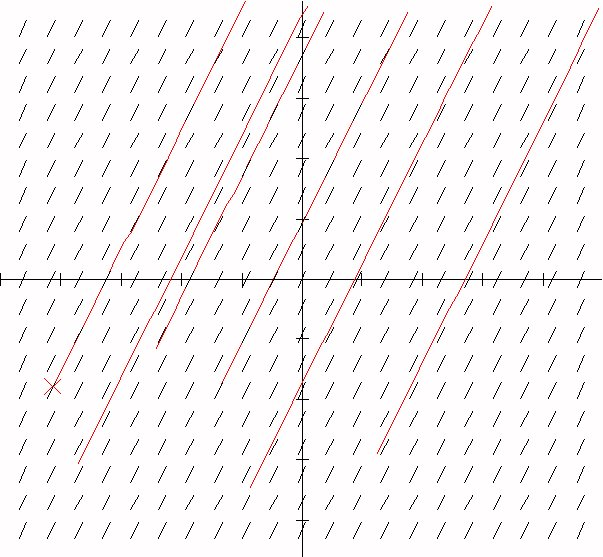1.  f : R2 -> R

2.

The end!
:)